انجمن را در گوگل محبوب کنيد :Artificial Intelligence - هوش مصنوعی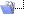پرولوگ، سورس کد الگوریتم کوتاهترین مسیر
 نام کاربري ذخيره؟ رمز عبور
 ثبت نام ارسال دعوتنامه به دوستان ! راهنماي سايت ليست كاربران Social Groups تقويم جستجو ارسالهاي امروز علامت گذاري بفرم خوانده شدهتبليغات سايت
 Iranian Association for the Advancement of Artificial Intelligence۰۵-۷-۱۳۹۲, ۰۶:۴۲ بعد از ظهر #1 (لینک دائم) عضو جدیدتاريخ عضويت: مرداد ۱۳۹۲ پست ها: 1 تشكرها: 14 0 تشكر در 0 پست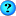پرولوگ، سورس کد الگوریتم کوتاهترین مسیر سلام و خسته نباشید خدمت همه ی دوستای خوبم برای درس منطق باید تا ی هفته دیگه ی پروژه پرولوگ با توضیحات تحویل بدم،راستش تا حالا اصلا پرولوگ کار نکردم،اگه بتونم این پروژه رو تحویل بدم دیگه هم باهاش کاری ندارم! الگوریتم کوتاهترین مسیر Dijkstra رو از اینترنت گیر اوردم اما ازش سر در نمیارم،prolog اینقد کامپایلر داره که حتی نمیدونم با چه کامپایلری اینو نوشتن اگه کسی از دوستان بتونه در مورد هر کدوم از بندهای این سورس کد ی توضیح هر چند اجمالی! بده واقعا ممنون میشم مرسی از همه عزیزان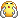************************************************** *********************** * A Modified Dijkstra algorithm * to determine one-to-all shortest paths in a directed unweighted graph * * A graph to analyze is specified by a list of its vertices and * by a set of adjacency lists, for each of the vertices. * For example, the following facts * vertices([a,b,c,d,e,f]). * adjacent(a,[b,d,f]). * adjacent(b,[c,f]). * specify a graph with 6 vertices and _directed_ edges a->b, a->d, * a->f, b->c, b->f. All edges are directed and have a weight ("length", * "cost", etc) of one. * * A predicate shortest_paths(a,ShPaths) would find all shortest paths * from a to all the other vertices of the graph (if accessible) and * return the list ShPaths of structures shortest_path(dest,path,length). * Each structure shortest_path(dest,path,lenth) describes a shortest * path from vertex 'a' to a vertex 'dest'. For example, * shortest_path(b,[b,c,a],3) * tells that the shortest path from 'a' to 'b' goes through 'c' * and has the length of 3. * * As all the edges have the same weight, an edge between two vertices * is the shortest path between these two vertices. Thus to find shortest * paths from a given vertex to the others we merely need to perform * a breadth-first search. * * Time complexity * For every vertex that is accessible from the origin we analyze * all its neighbors in the adjacency list. We thus look at every directed edge * in the graph, at most once. Therefore, the time complexity is * O(e), e being the no. of (directed) edges. * ************************************************** *********************** */ /* Given below is an example of the graph */ /* to work with */ vertices([a,b,c,d,e,f]). adjacent(a,[b,d,f]). adjacent(b,[c,f]). adjacent(c,[d]). adjacent(d,[]). adjacent(e,[f,d]). adjacent(f,[d]). /* *------------------------------------------------------------------------ * Root predicate * Note, that the global data base is dynamically updated with the * following facts * to_handle(x). * This fact tells a name of a vertex to handle. At first * it is the vertex-origin. Once a vertex is handled, all its * neighbors will have to be handled in turn. Note, to_handle(x) * facts have to form a queue: the facts are picked for processing * from the top, new facts are added at the bottom. * This guarantees the breadth-first search. * free(x). * This fact tells a vertex a shortest path to which * hasn't been determined yet. * shortest_path(x,[x,y,...],size). * gives the shortest path from the Origin to x * once established. * */ shortest_path(A,ShPaths) :- /* Make all the vertices but the initial free */ vertices(Vertices), make_free(Vertices), retract(free(A)), assert(shortest_path(A,[A],0)), assert(to_handle(A)), /* Start with A */ loop, setof(shortest_path(X,L,D),shortest_path(X,L,D),Sh Paths). make_free([]). make_free([X|Y]) :- assert(free(X)), make_free(Y). loop :- /* Handling the vertices, i.e. extend the path */ retract(to_handle(A)), adjacent(A,AdjList), nl,write('Handling the vertex '),write(A),nl, handle(A,AdjList), loop. loop. /* Extend the path from the Origin through A */ handle(A,[]). handle(A,[B|Y]) :- retract(free(B)), shortest_path(A,Path,Dist), /* Path that has lead to A from origin*/ Dist1 is Dist + 1, assert(shortest_path(B,[B|Path],Dist1)), /* Extend the path to B */ write(' extending to '),write(B),nl, assertz(to_handle(B)), /* add to the bottom of queue */ handle(A,Y). /* And handle other neighbors */ handle(A,[_|Y]) :- handle(A,Y). /* If B wasn't free */ % Try it out... ?- print('all shortest pathes from a vertex a'),shortest_path(a,ShPaths), print(ShPaths).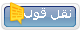#ADS نشان دهنده تبلیغات تبليغگرتاريخ عضويت: - محل سكونت: - سن: 2010 پست ها: -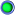Tags prolog, درخواست کمک،shortest pathكاربران در حال ديدن تاپيک: 1 (0 عضو و 1 مهمان)
 ابزارهاي تاپيکنمايش به صورت چاپگرارسال اين صفحه به ايميل نحوه نمايشLinear ModeSwitch to Hybrid ModeSwitch to Threaded Modeقوانين ارسال You may not post new threads You may not post replies You may not post attachments You may not edit your posts BB code is فعال شکلکها فعال است كد [IMG] فعال است كدهاي HTML غير فعال استTrackbacks are فعال Pingbacks are فعال Refbacks are فعال Forum Rules

زمان محلي شما با تنظيم GMT +3.5 هم اکنون ۰۹:۰۲ بعد از ظهر ميباشد.

 -- English (US) -- Farsi تماس با ما - هوش مصنوعی - آرشيو - بالا